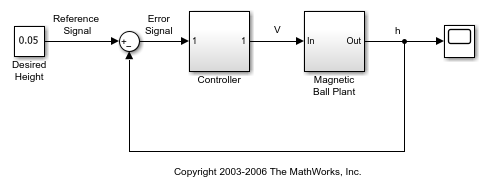# operpoint

Create operating point for Simulink model

## Syntax

``op = operpoint(mdl)``

## Description

example

````op = operpoint(mdl)` returns the operating point of Simulink® model `mdl`. You can compute a linear model of your system at this operating point using the `linearize` function.```

## Examples

collapse all

```open_system('magball') ```Create operating point for the model.

```op = operpoint('magball') ```
``` Operating point for the Model magball. (Time-Varying Components Evaluated at time t=0) States: ---------- (1.) magball/Controller/PID Controller/Filter/Cont. Filter/Filter x: 0 (2.) magball/Controller/PID Controller/Integrator/Continuous/Integrator x: 14 (3.) magball/Magnetic Ball Plant/Current x: 7 (4.) magball/Magnetic Ball Plant/dhdt x: 0 (5.) magball/Magnetic Ball Plant/height x: 0.05 Inputs: None ---------- ```

`op` lists each block in the model that has states. There are no root-level inports in this model, therefore `op` does not contain inputs.

You can create new operating-point variables in three ways:

• Using the `operpoint` function

• Using assignment with the equals (`=`) operator

• Using the `copy` function

Using the `=` operator results in linked variables that both point to the same underlying data. Using the `copy` function results in an independent operating-point object. In this example, create operating-point objects both ways, and examine their behavior.

```mdl = 'watertank'; open_system(mdl); op1 = operpoint(mdl)```
``` Operating point for the Model watertank. (Time-Varying Components Evaluated at time t=0) States: ---------- (1.) watertank/PID Controller/Integrator/Continuous/Integrator x: 0 (2.) watertank/Water-Tank System/H x: 1 Inputs: None ---------- ```

Create a new operating-point object using assignment with the `=` operator.

`op2 = op1;`

`op2` is an operating-point object that points to the same underlying data as `op1`. Because of this link, you cannot independently change properties of the two operating-point objects. To see this, change a property of `op2`. For instance, change the value for the first state from 0 to 2. The change shows in the `States` section of the display.

`op2.States(1).x = 2`
``` Operating point for the Model watertank. (Time-Varying Components Evaluated at time t=0) States: ---------- (1.) watertank/PID Controller/Integrator/Continuous/Integrator x: 2 (2.) watertank/Water-Tank System/H x: 1 Inputs: None ---------- ```

Examine the display of `op1` to see that the corresponding property value of `op1` also changes from 0 to 2.

`op1`
``` Operating point for the Model watertank. (Time-Varying Components Evaluated at time t=0) States: ---------- (1.) watertank/PID Controller/Integrator/Continuous/Integrator x: 2 (2.) watertank/Water-Tank System/H x: 1 Inputs: None ---------- ```

To create an independent copy of an operating-point object, use the `copy` function.

`op3 = copy(op1);`

Now, when you change a property of `op3`, `op1` does not change. For instance, change the value for the first state from 2 to 4.

`op3.States(1).x = 4`
``` Operating point for the Model watertank. (Time-Varying Components Evaluated at time t=0) States: ---------- (1.) watertank/PID Controller/Integrator/Continuous/Integrator x: 4 (2.) watertank/Water-Tank System/H x: 1 Inputs: None ---------- ```

In `op1`, the corresponding value remains 2.

`op1.States(1).x`
```ans = 2 ```

This copy behavior occurs because the operating-point object is a handle object. For more information about handle objects, see Handle Object Behavior (MATLAB).

## Input Arguments

collapse all

Simulink model name, specified as a character vector or string. The model must be in the current working folder or on the MATLAB® path.

## Output Arguments

collapse all

Operating point, returned as an operating-point object with the following properties.

PropertyDescription
`Model`Simulink model name, returned as a character vector.
`States`

State operating point, returned as a vector of state objects. Each entry in `States` represents the supported states of one Simulink block.

For a list of supported states for operating point objects, see Simulink Model States Included in Operating Point Object.

### Note

If the block has multiple named continuous states, `States` contains one structure for each named state.

Each state object has the following fields:

FieldDescription
`Nx` (read only)

Number of states in the block

`Block`

Block path, returned as a character vector.

`StateName`

State name

`x`

Values of all supported block states, returned as a vector of length `Nx`.

`Ts`

Sample time and offset of each supported block state, returned as a vector. For continuous-time systems, `Ts` is zero.

`SampleType`

State time rate, returned as one of the following:

• `'CSTATE'` — Continuous-time state

• `'DSTATE'` — Discrete-time state

`inReferencedModel`

Flag indicating whether the block is inside a reference model, returned as one of the following:

• `1` — Block is inside a reference model.

• `0` — Block is in the current model file.

`Description`

Block state description, returned as a character vector.

`Inputs`

Input level at the operating point, returned as a vector of input objects. Each entry in `Inputs` represents the input levels of one root-level inport block in the model.

Each input object has the following fields:

FieldDescription
`Block`

Inport block name

`PortWidth`

Number of inport block signals

`PortDimensions`

Dimension of signals accepted by the inport

`u`

Inport block input levels at the operating point, returned as a vector of length `PortWidth`.

`Description`

Inport block input description, returned as a character vector.

`Time`

Times at which any time-varying functions in the model are evaluated, returned as a vector.

`Version`

Object version number

## Tips

• You can create new `operpoint` variables of in three ways:

• Construct a new object with the `operpoint` command.

• Create a new variable by assignment with the equals (=) operator.

• Copy an `operpoint` object using the `copy` command.

Using `operpoint` or `copy` creates a new, independent object. When you use assignment, there is a link between the old and new variable. For an example, see Copy an Operating Point.

## Alternative Functionality

The `operpoint` function returns an operating point with the current state and input values of the model. To create an operating point that meets your application specifications, use the `findop` function. For more information, see Compute Steady-State Operating Points.